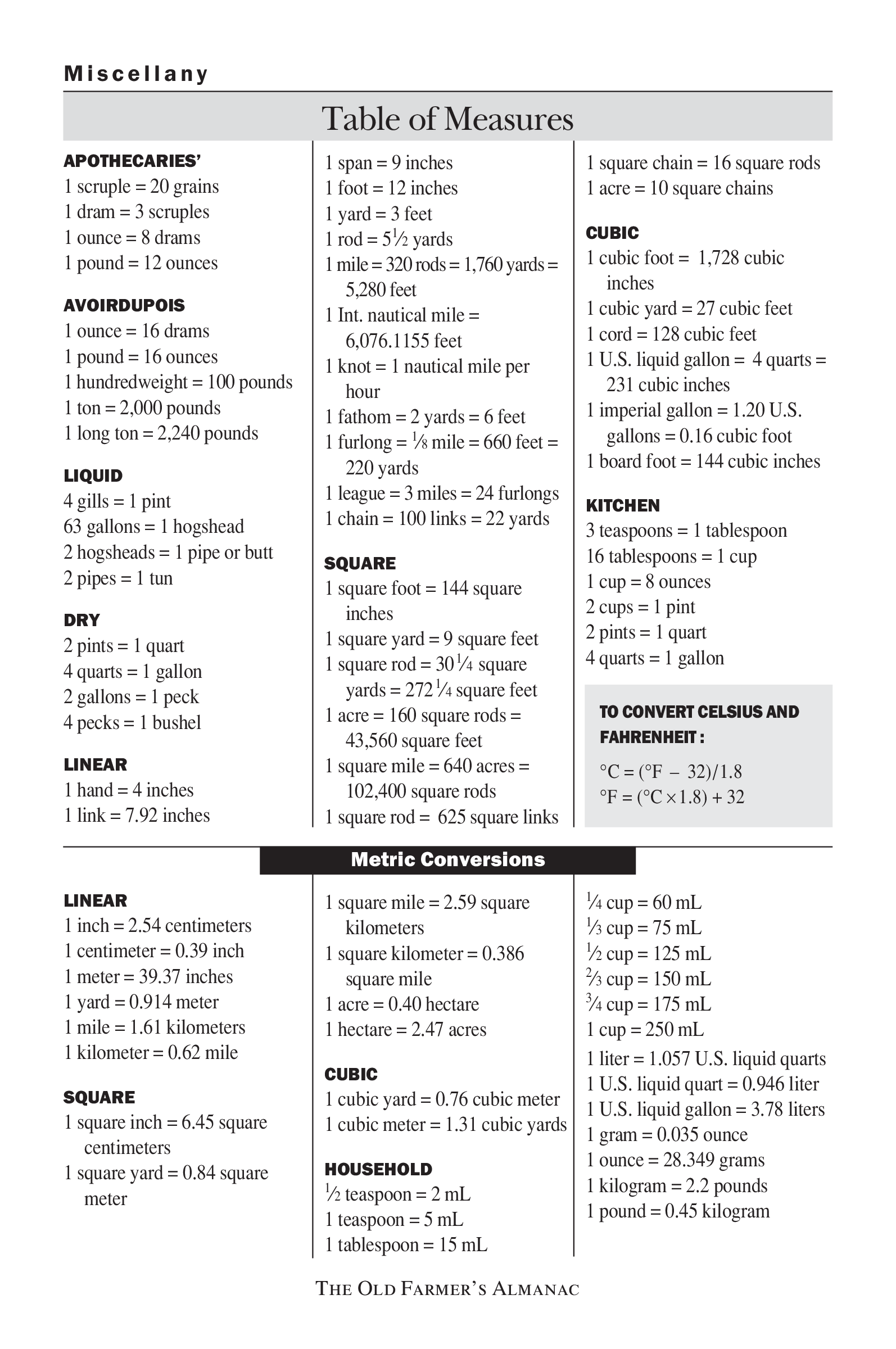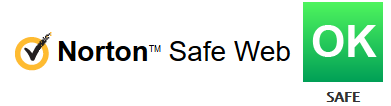# Liquid Measurement Equivalent Chart

Save, fill-In The Blanks, Print, Done!Click on image to zoom / Click button below to see more images

• This Document Has Been Certified by a Professional
• 100% customizable
• Language: English

ABT template rating: 8

Malware- and virusfree. Scanned by:How to draft a Liquid Measurement Equivalent Chart? An easy way to start completing your document is to download this Liquid Measurement Equivalent Chart template now!

Every day brings new projects, emails, documents, and task lists, and often it is not that different from the work you have done before. Many of our day-to-day tasks are similar to something we have done before. Don't reinvent the wheel every time you need to make measurement calculations.

The conversion measurement template provides info regarding the following:

Nautical mile = 6,076.1155
feet 1 knot = 1 nautical mile per hour 1 fathom = 2 yards = 6 feet 1 furlong = 1⁄ 8 mile = 660 feet = 220 yards 1 league = 3 miles = 24 furlongs 1 chain = 100 links = 22 yards SQUARE 1 square foot = 144 square inches 1 square yard = 9 square feet 1 square rod = 30 1⁄ 4 square yards = 272 1⁄ 4 square feet 1 acre = 160 square rods = 43,560 square feet 1 square mile = 640 acres = 102,400 square rods 1 square rod = 625 square links 1 square chain = 16 square rods 1 acre = 10 square chains CUBIC 1 cubic foot = 1,728 cubic inches 1 cubic yard = 27 cubic feet 1 cord = 128 cubic feet 1 U.S. liquid gallon = 4 quarts = 231 cubic inches 1 imperial gallon = 1.20 U.S. gallons = 0.16 cubic foot 1 board foot = 144 cubic inches KITCHEN 3 teaspoons = 1 tablespoon 16 tablespoons = 1 cup 1 cup = 8 ounces 2 cups = 1 pint 2 pints = 1 quart 4 quarts = 1 gallon

TO CONVERT CELSIUS AND FAHRENHEIT : °C = (°F – 32)/1.8 °F = (°C × 1.8) + 32 Metric Conversions

LINEAR 1 inch = 2.54 centimeters 1 centimeter = 0.39 inch 1 meter = 39.37 inches 1 yard = 0.914 meter 1 mile = 1.61 kilometers 1 kilometer = 0.62 mile SQUARE 1 square inch = 6.45 square centimeters 1 square yard = 0.84 square meter 1 square mile = 2.59 square kilometers 1 square kilometer = 0.386 square mile 1 acre = 0.40 hectare 1 hectare = 2.47 acres 1 ⁄ 4 cup = 60 mL ⁄ 3 cup = 75 mL 1 ⁄ 2 cup = 125 mL 2 ⁄ 3 cup = 150 mL 3 ⁄ 4 cup = 175 mL 1 cup = 250 mL 1 liter = 1.057 U.S. liquid quarts CUBIC 1 U.S. liquid quart = 0.946 liter 1 cubic yard = 0.76 cubic meter 1 U.S. liquid gallon = 3.78 liters 1 cubic meter = 1.31 cubic yards 1 gram = 0.035 ounce 1 ounce = 28.349 grams HOUSEHOLD 1 kilogram = 2.2 pounds 1 ⁄ 2 teaspoon = 2 mL 1 pound = 0.45 kilogram 1 teaspoon = 5 mL 1 tablespoon = 15 mL The Old Farmer’s Almanac 1.
Instead, we provide this standardized Liquid Measurement Equivalent Chart template with text and formatting as a starting point to help you do the conversion or find the equivalent. If time or quality is of the essence, this ready-made template can help you to save time and to focus on the topics that really matter!

Using this chart template guarantees you will save time, cost, and effort! It comes in Microsoft Office format, is ready to be tailored to your personal needs. Completing your chart has never been easier! If you wish to convert Celcius to Fahrenheit, have a look at this C to F temperature conversion chart.

DISCLAIMER
Nothing on this site shall be considered legal advice and no attorney-client relationship is established.